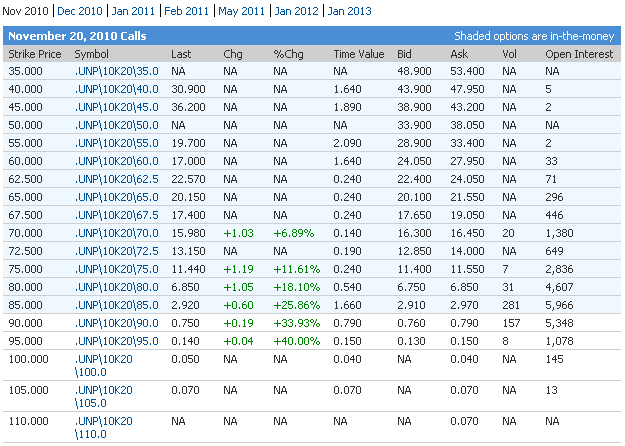Option prices can be decomposed into the option’s intrinsic value and time value.

### Intrinsic Value

Intrinsic value is the amount that an option is immediately worth given the relation between the option striking price and the current stock price

Intrinsic value is the payoff value of the option were it possible to exercise immediately; if out of the money, zero, otherwise the difference between the strike and current stock price.

Example:

Let us look at the option prices of Union Pacific Corp. The closing price of UNP was 86.31.The November 2010, $40 strike call is selling for$47.950. The closing UNP price was $86.31.47. So$86.31-$40.00=$46.31 is the intrinsic value of the option.

For a call option, intrinsic value = Stock price – Striking price

For a put option, intrinsic value = Striking price – Stock price